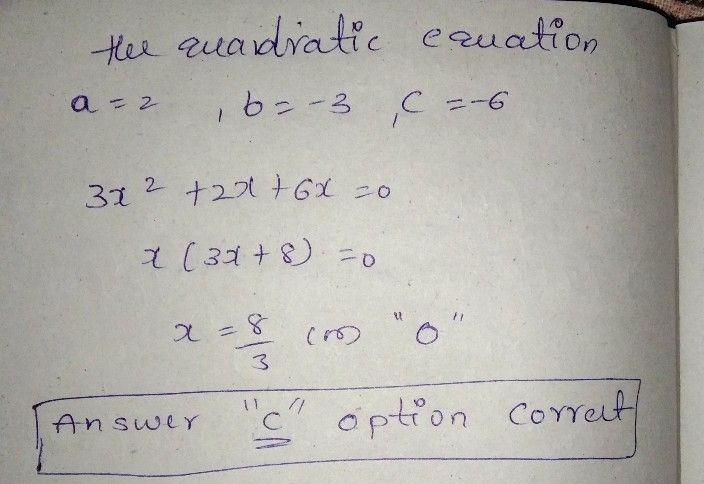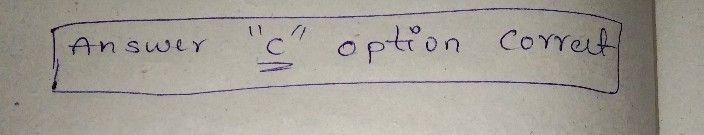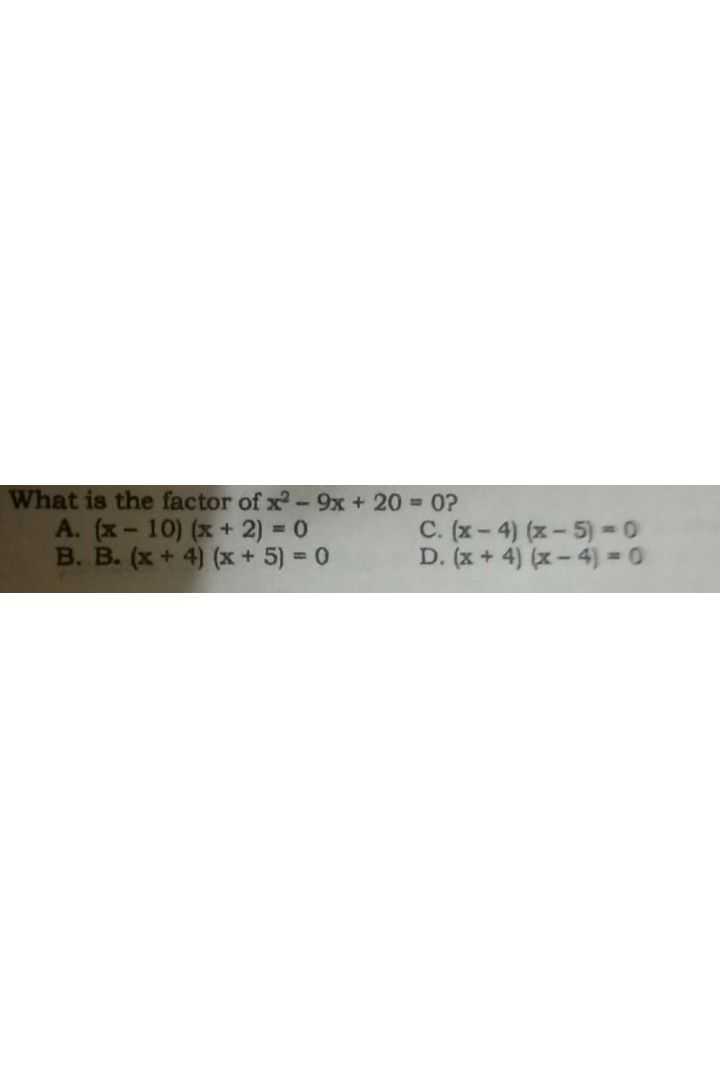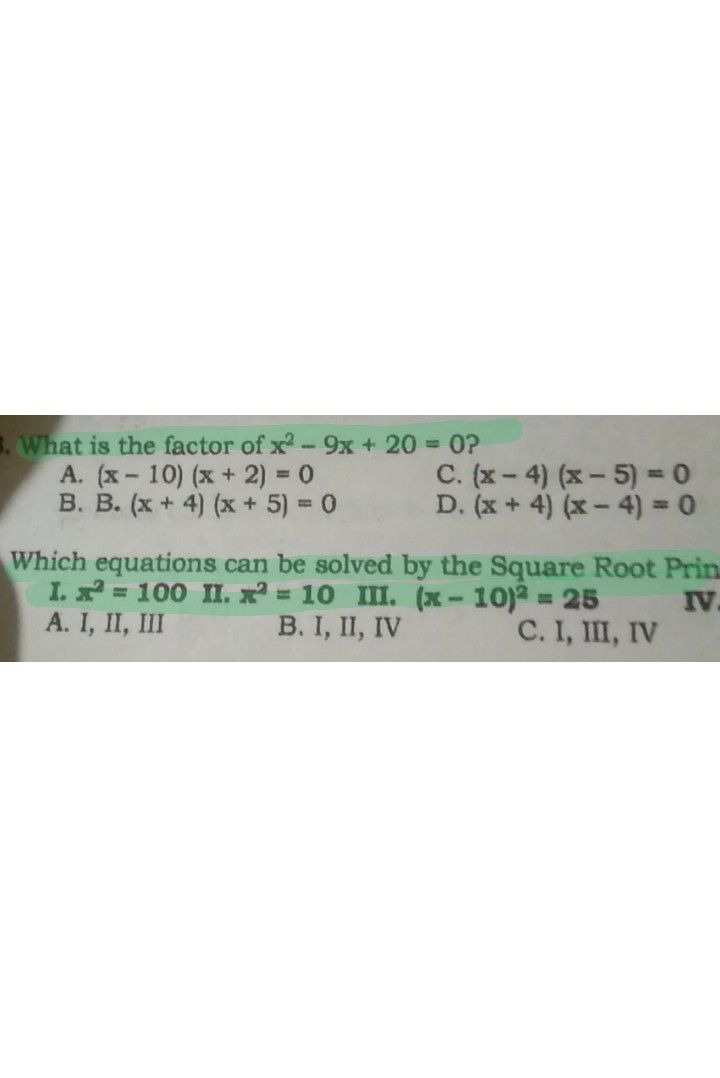Symbol
ProblemWhat is the quadratic equation if $a=2$ $b=-3$ and $=-6$ $A$ $x^{2}-3x-6=0$ C. $3x^{2}+2x+6x=0$ B. B. $2x^{2}-3x-6=0$ $1D$ $2x^{2}+3x+6$ $+6=0$